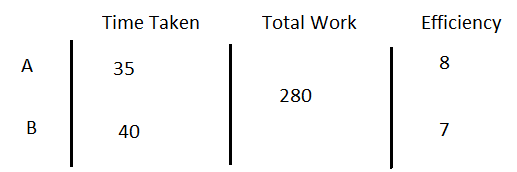Question of The Day30-05-2022

A and B can do a piece of work in 35 and 40 days respectively. They started the work together but A left the work after some time and B finished the remaining work in 23 days. Find out after how many days A left the work (approx.)?

Correct Answer : a ) 8

Explanation :

Basic Method:

Time Taken by A to complete the work = 35 days

Work done by A in 1 day = $$1 \over 35$$ units

Time Taken by B to complete the work = 40 days

Work done by B in 1 day = $$1\over40$$ units

Work done by A and B together in 1 day (Efficiency of A and B together):

$$1 \over 35$$+ $$1\over40$$= $$15\over280$$

B work alone for 23 days

Work done by B in 23 days= $$23 \over 40$$ units

Assume the complete work being done is 1

Work done by A and B together= 1- $$23 \over 40$$=$$17 \over 40$$

Time taken to complete the work= $$Total work \over Efficiency$$

Time taken by A and B to do 17/40 units of work = $${{17\over40}\over{15\over280}}={{17*280}\over{40*15}}$$

⇒ 7.9333 days (Approx. ≈8 days)

Therefore, A left the work after 8 days.

Alternative Method:

Time Taken by A to complete the work = 35 days

Time Taken by B to complete the work = 40 days

Let’s assume total work done by A and B = LCM (35, 40) = 280

Work done by A in 1 day= $$280 \over 35$$=8 units

Time Taken by B to complete the work = 40 days

Work done by B in 1 day= $$280\over 40$$=7 unitsB work alone for 23 days

Work done by B alone = 23*7 = 161

Remaining work done by A and B together = 280-161 = 119

Work done by A and B together in 1 day = 8 + 7 = 15 units

Time taken by A and B to do the remaining work:

⇒$$119 \over 15$$=7.93333..     (Approx. ≈8)

Therefore, A left the work after 8 days.

Hence, (a) is the correct answer.0